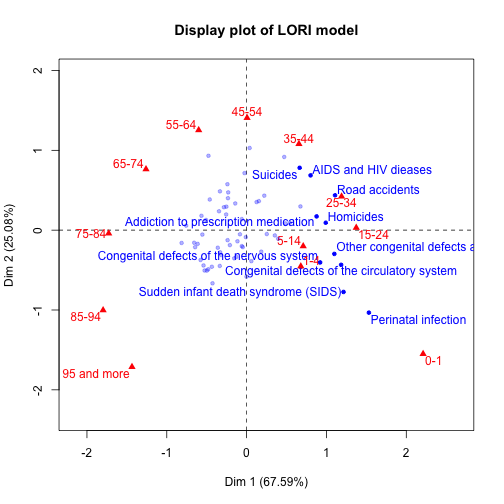## Regularized log-bilinear model

This vignette explains how to use the package lori to fit a regularized log-bilinear model for denoising and visualizing count data. Let $$Y\in\mathbb{R}^{n\times p}$$ be a contingency table modeled as Poisson variables. Let $$X\in\mathbb{R}^{n\times p}$$ be a parameter matrix such that for all $$1\leq i\leq n$$ and $$1\leq j\leq p$$, and denoting $$\mathcal{P}(l)$$ the Poisson distribution of parameter $$l$$: $Y_{ij}|X_{ij} \sim \mathcal{P}(\mathrm{e}^X_{ij}).$ The log-bilinear model assumes the following decomposition for $$X_{ij}$$: $X_{ij} = \mu + \alpha_i + \beta_j + \Theta_{ij},$ where $$\mu$$ is an offset, $$\alpha_i$$ and $$\beta_j$$ are main row and column effects, and $$\Theta_{ij}$$ is an interaction term. When the matrix $$\Theta$$ is constrained to have a fixed rank $$K$$, $X_{ij} = \mu + \alpha_i + \beta_j + \Theta_{ij},\quad \text{rank}(\Theta) = K$ is called the RC($$K$$) model. We consider a variant of the log-bilinear model where the rank constraint $$\text{rank}(\Theta) = K$$ is replaced by a convex relaxation of with a nuclear norm penalty of $$\Theta$$. Our estimator is the following. $(\hat\mu, \hat\alpha,\hat\beta,\hat\Theta) = \text{argmin}_{\mu, \alpha,\beta,\Theta} \Phi(\mu, \alpha,\beta,\Theta) + \gamma\left\|\Theta\right\|_*,\ s.t.\quad \sum_{i=1}^n\alpha_i = 0, \sum_{j=1}^p\beta_j = 0, \sum_{i,j}\Theta_{ij} = 0,$ where $$\Phi$$ is the Poisson log-likelihood $\Phi(\mu, \alpha,\beta,\Theta) = \sum_{i=1}^n\sum_{j=1}^p -Y_{ij}X_{ij} + \exp(X_{ij}),$ and $$\lambda$$ is a regularization parameter acting as a trade-off between fitting a data and having a low rank. We refer to this method as Low-rank Interaction (LORI). Such regularization can prove useful when counts are large, $$Y$$ contains many $$0$$ values or is of large dimensions, situations where classical implementations of the RC($$K$$) model might fail.

## Mortality data

We illustrate this model on the contingency table \textit{Death}, available at \url{http://factominer.free.fr/book/death.csv} and described in \citet{paghuss2010da}. It crosses causes of death with age categories for the French population in the year 2006. Age is encoded as a categorical variable with 12 categories ($$0-1$$, $$1-4$$, $$5-14$$, etc.) and $$65$$ possible mortality causes are considered. A cell of the table therefore contains the number of people who died from a particular cause in a particular age category during the year 2006. The LORI method is applied on this dataset.

library(lori)
library(FactoMineR)
library(logmult)

## Loading required package: gnm

##
## Attaching package: 'logmult'

## The following object is masked from 'package:gnm':
##
##     se

death <- read.table("http://factominer.free.fr/book/death.csv", header = TRUE,
sep = ";", row.names = 1)
colnames(death) <- c("0-1", "1-4", "5-14", "15-24", "25-34", "35-44", "45-54", "55-64", "65-74",
"75-84", "85-94", "95 and more")
## Extract first 65 rows correponding to year 2006
death <- death[66:130,]
rownames(death) <- sapply(rownames(death), function(s) gsub("^.*?_","",s))
n <- nrow(death)
p <- ncol(death)

##  "computing empirical distribution..."
##  "starting optimization..."
##  "iteration: 1000"
##  "objective step: -3.10931682179216e-07"
##  "iteration: 2000"
##  "objective step: -9.44337443797849e-08"
##  "iteration: 3000"
##  "objective step: -3.79213815904222e-08"
##  "iteration: 4000"
##  "objective step: -2.73148543783464e-08"
##  "iteration: 5000"
##  "objective step: -8.49831849336624e-09"
##  "iteration: 6000"
##  "objective step: -4.25461621489376e-09"
##  "iteration: 7000"
##  "objective step: -1.27874955069274e-09"
##  "iteration: 8000"
##  "objective step: -4.7202775022015e-10"
##  "iteration: 9000"
##  "objective step: -1.84627424459904e-10"
##  "iteration: 10000"
##  "objective step: -7.27595761418343e-11"
##  "model converged !"


We represent biplot visualizations of the data in the two first dimensions of interaction, keeping only the 10 death causes that have the highest contributions in the first two dimensions. Models such as log-bilinear models provide a distance interpretation as follows. Two age categories or two mortality causes that are close to one another have similar profiles in the contingency table whereas an age category and a mortality cause that are close interact highly. The shape of the biplot and in particular the structure of the age categories (in red) is known as the Guttman or horseshoe effect.

## Two dimensional display plot

plot_interaction(theta_lori, title = "Display plot of LORI model", selectRow = "contrib 10")